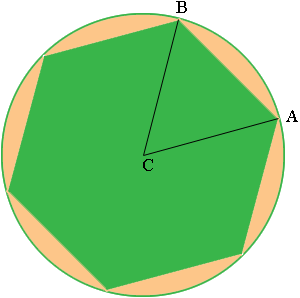SEARCH HOMEMath Central Quandaries & QueriesQuestion from Courtney, a student: If ABCDEF is a regular hexagon inscribed in a circle of radius r, prove that the length of each side of the hexagon equals r.Hi Courtney,

Did you draw a diagram?I drew two radii to vertices of the hexagon. Draw all 6. What is the measure of the angle BCA? What are the lengths of BC nd CA? What does this tell you about the angles ABC and CAB? What are the measures of these angles? What is the length of AB?

PennyMath Central is supported by the University of Regina and The Pacific Institute for the Mathematical Sciences.